# 简述

椭圆曲线密码学，简称ECC。是一种建立公开加密的算法，也就是非对称加密。和RSA类似。被公认在给定密钥长度下最安全的加密算法。应用范围很广，主要的三个技术TLS、PGP、SSH都在使用它，特别是以BTC为代表的数字货币。

# 椭圆曲线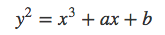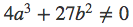a和b决定了曲线在坐标系的不同形状。举个例子：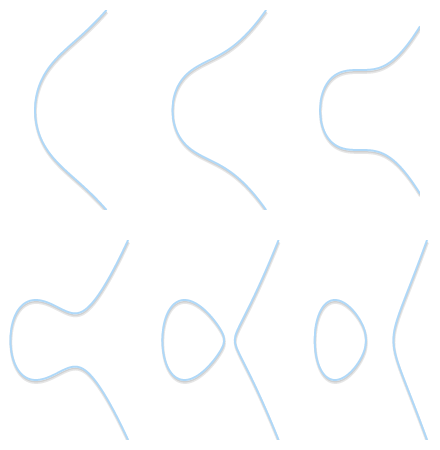# 阿贝尔群

• 封闭性：如果a和b都是群成员，那么a+b也是群成员。
• 组合性：(a+b)+c=a+(b+c)
• 单位元：存在确切的一个值可以保证 a+0=0+a=a成立，我们称之为单位元
• 逆元：每个成员都有一个相反数：对于任意值a必定存在b使得a+b=0

• 封闭性：a、b属于整数，a+b也属于整数
• 组合性：(a+b)+c=a+(b+c)
• 单位元：0值就是单位元
• 逆元：a的逆元就是-a

# 椭圆曲线的加法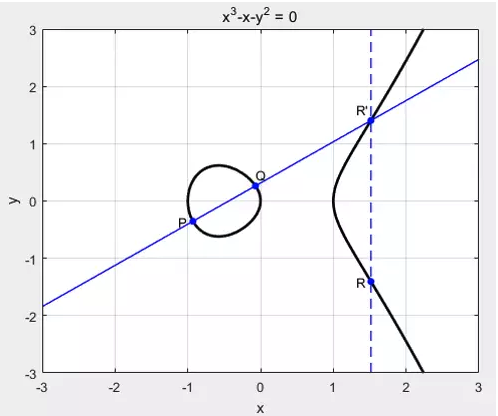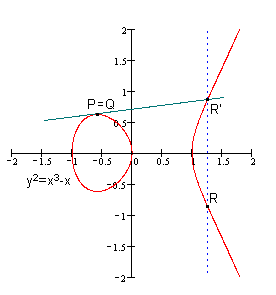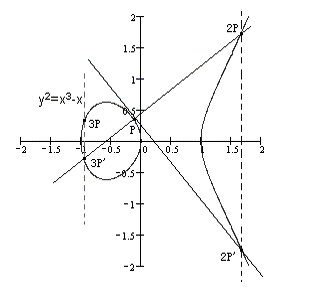# 椭圆曲线上的离散对数问题

• 椭圆曲线E
• 椭圆曲线E上的一点G(基点)
• 椭圆曲线E上的一点xG(x倍的G)

• x

# 有限域上的椭圆曲线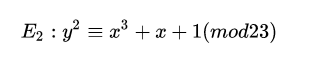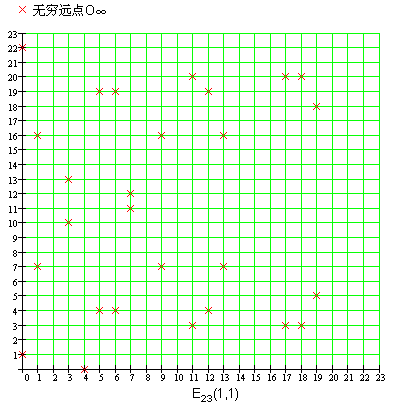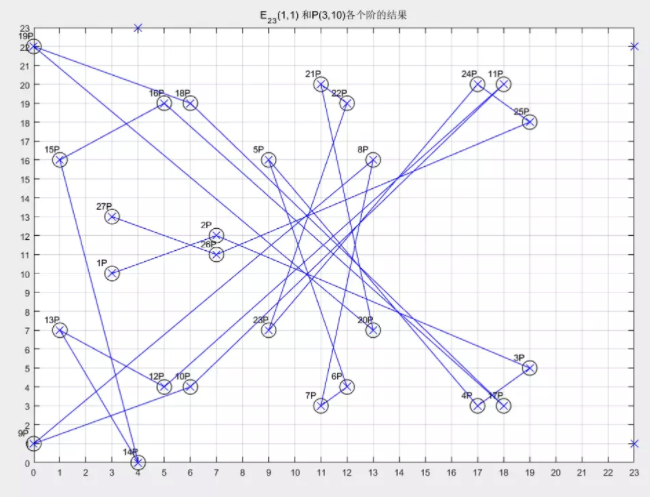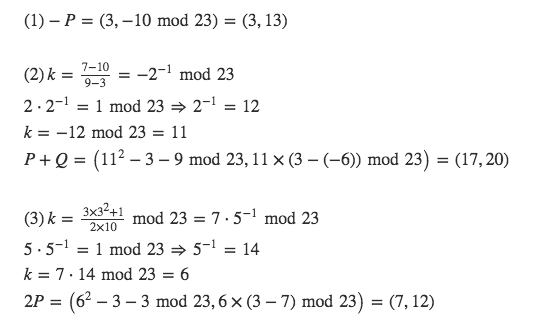# 椭圆曲线加密ECC

• Alice选定一条椭圆曲线E，并取椭圆曲线上一点作为基点G 假设选定E29(4,20)，基点G(13,23) , 基点G的阶数n=37
• Alice选择一个私有密钥k（k<n），并生成公开密钥K=kG 比如25, K= kG = 25G = (14,6）
• Alice将E和点K、G传给Bob
• Bob收到信息后，将待传输的明文编码到上的一点M（编码方法略），并产生一个随机整数r（r<n,n为G的阶数） 假设r=6 要加密的信息为3,因为M也要在E29(4,20) 所以M=(3,28)
• Bob计算点C1=M+rK和C2=rG C1= M+6K= M+6*25*G=M+2G=(3,28)+(27,27)=(6,12) C2=6G=(5,7)
• Bob将C1、C2传给Alice
• Alice收到信息后，计算C1-kC2，结果就应该是点M C1-kC2 =(6,12)-25C2 =(6,12)-25*6G =(6,12)-2G =(6,12)-(27,27) =(6,12)+(27,2) =(3,28)

## 参数要求：

• p越大安全性越好，但会导致计算速度变慢,200bit左右可满足一般安全要求
• n应为质数

# ECC VS  RSA

## 优点：

• 安全性能更高
• 160位ECC与1024位RSA、DSA有相同的安全强度
• 处理速度更快
• 在私钥的处理速度上，ECC远 比RSA、DSA快得多
• 带宽要求更低
• 存储空间更小
• ECC的密钥尺寸和系统参数与RSA、DSA相比要小得多

## 缺点：

• 设计困难，实现复杂
• 如果序列号设计过短，那么安全性并没有想象中的完善

# 椭圆曲线签名算法

## 私钥签名：

• Alice选择随机数r，计算点rG(x, y)。
• Alice根据随机数r、消息M的哈希h、私钥k，计算s = (h + kx)/r。
• Alice将消息M、和签名{rG, s}发给接收方。

## 公钥验证签名：

• Bob收到消息M、以及签名{rG=(x,y), s}。
• Bob根据消息M,求哈希h。
• 使用发送方公钥K计算：hG/s + xK/s，并与rG比较，如相等即验签成功。

## 验证原理

hG/s + xK/s = hG/s + x(kG)/s = (h+xk)G/s = r(h+xk)G / (h+kx) = rG

09-261万+

#### ECC椭圆曲线加解密原理详解(配图)09-06474

#### ECDSA椭圆曲线数字签名算法概要和使用说明

03-011427

#### 椭圆曲线数字签名算法（ECDSA）©️2020 CSDN 皮肤主题: 编程工作室 设计师: CSDN官方博客点击重新获取扫码支付1.余额是钱包充值的虚拟货币，按照1:1的比例进行支付金额的抵扣。
2.余额无法直接购买下载，可以购买VIP、C币套餐、付费专栏及课程。余额充值